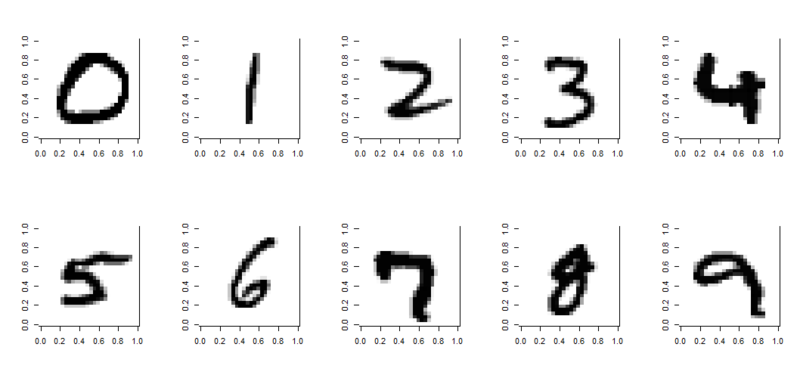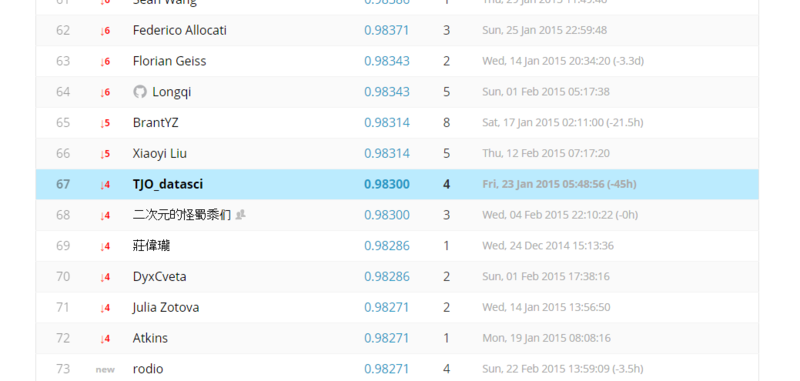# Deep Learning with {h2o} on MNIST dataset (and Kaggle competition)

In the previous post we saw how Deep Learning with {h2o} works and how Deep Belief Nets implemented by h2o.deeplearning draw decision boundaries for XOR patterns.

Of course entirely the same framework can be applied to other general and usual datasets - including Kaggle competitions. For just a curiosity, I were looking for a free MNIST dataset and fortunately I found Kaggle provides it as below.

I know Convolutional NN (ConvNet or CNN) better works for such a 2D image classification task than Deep Belief Net... there are some well-known and well-established libraries such as Caffe, CUDA-ConvNet, Torch7, etc., but they may take a little more to implement for (lazy) me. Here I ran a brief and quick trial with a MNIST dataset for h2o.deeplearning in order to check its performance.

### MNIST dataset from Kaggle

Our first mission here is to try h2o.deeplearning briefly, so let's divide it into a train and test dataset. MNIST dataset has 10 categories of dependent variables and we have to divide them with balancing all of 10 categories.

```> dat<-read.csv("train.csv", header=TRUE)
> labels<-dat[,1]
> test_idx<-c()
> for (i in 1:10) {
+ 	tmp1<-which(labels==(i-1))
+ 	tmp2<-sample(tmp1,1000,replace=F)
+ 	test_idx<-c(test_idx,tmp2)
+ }
> test<-dat[test_idx,]
> train<-dat[-test_idx,]
> write.table(train,file="prac_train.csv",quote=F,col.names=T,row.names=F,sep=",")
> write.table(test,file="prac_test.csv",quote=F,col.names=T,row.names=F,sep=",")
```

Now we have a customized dataset with "prac_train.csv" and "prac_test.csv" files. By the way, if you are unwilling to prepare the dataset by yourself, I uploaded them on my GitHub repository. You can get them from there.

Please note that this dataset is a little heavier so you'll take more time to download than expected.

Just for visualization, we can draw each digit in R. Please try as below (sorry for my ugly code...).

```> test<-read.delim("prac_test.csv",sep=',')
> id0<-which(test\$label==0)
> id1<-which(test\$label==1)
> id2<-which(test\$label==2)
> id3<-which(test\$label==3)
> id4<-which(test\$label==4)
> id5<-which(test\$label==5)
> id6<-which(test\$label==6)
> id7<-which(test\$label==7)
> id8<-which(test\$label==8)
> id9<-which(test\$label==9)
> par(mfrow=c(2,5))
> image(t(apply(matrix(as.vector(as.matrix(test[id0,-1])),ncol=28,nrow=28,byrow=T),2,rev)),col=grey(seq(1,0,length.out=256)))
> image(t(apply(matrix(as.vector(as.matrix(test[id1,-1])),ncol=28,nrow=28,byrow=T),2,rev)),col=grey(seq(1,0,length.out=256)))
> image(t(apply(matrix(as.vector(as.matrix(test[id2,-1])),ncol=28,nrow=28,byrow=T),2,rev)),col=grey(seq(1,0,length.out=256)))
> image(t(apply(matrix(as.vector(as.matrix(test[id3,-1])),ncol=28,nrow=28,byrow=T),2,rev)),col=grey(seq(1,0,length.out=256)))
> image(t(apply(matrix(as.vector(as.matrix(test[id4,-1])),ncol=28,nrow=28,byrow=T),2,rev)),col=grey(seq(1,0,length.out=256)))
> image(t(apply(matrix(as.vector(as.matrix(test[id5,-1])),ncol=28,nrow=28,byrow=T),2,rev)),col=grey(seq(1,0,length.out=256)))
> image(t(apply(matrix(as.vector(as.matrix(test[id6,-1])),ncol=28,nrow=28,byrow=T),2,rev)),col=grey(seq(1,0,length.out=256)))
> image(t(apply(matrix(as.vector(as.matrix(test[id7,-1])),ncol=28,nrow=28,byrow=T),2,rev)),col=grey(seq(1,0,length.out=256)))
> image(t(apply(matrix(as.vector(as.matrix(test[id8,-1])),ncol=28,nrow=28,byrow=T),2,rev)),col=grey(seq(1,0,length.out=256)))
> image(t(apply(matrix(as.vector(as.matrix(test[id9,-1])),ncol=28,nrow=28,byrow=T),2,rev)),col=grey(seq(1,0,length.out=256)))
```As well known, a limited part of MNIST digits cannot be correctly identified even by human eyes, so in general it's said that 100 % accuracy is impossible.

### Run h2o.deeplearning: trial and error with tuning hyper parameters

Prior to trying h2o.deeplearning on MNIST dataset, first we have to boot {h2o} instance. Please remember how to boot it and set parameters required.

```> library(h2o)
> localH2O <- h2o.init(ip = "localhost", port = 54321, startH2O = TRUE, nthreads=-1)
> trData<-h2o.importFile(localH2O,path = "prac_train.csv")
> tsData<-h2o.importFile(localH2O,path = "prac_test.csv")
```

In order to set a benchmark, we run a random forest classifier first as MNIST Kaggle competition recommends.

```> prac_train <- read.csv("prac_train.csv")
> library(randomForest)
> prac_train\$label<-as.factor(prac_train\$label)
> prac.rf<-randomForest(label~.,prac_train)
> table(prac_test\$label,predict(prac.rf,newdata=prac_test[,-1]))

0   1   2   3   4   5   6   7   8   9
0 984   0   1   0   0   3   3   1   7   1
1   0 984   4   3   2   0   2   3   1   1
2   2   2 958   5   3   2   5   8  12   3
3   2   2  17 947   1   8   2   7  10   4
4   1   2   2   0 976   0   3   3   1  12
5   5   2   0  16   0 957   8   0   8   4
6   4   0   1   0   2   6 984   0   3   0
7   0   3   8   1   4   0   0 971   3  10
8   3   3   6   7   9   9   6   0 944  13
9   5   0   4  11  11   2   1  15   8 943

> sum(diag(table(prac_test\$label,predict(prac.rf,newdata=prac_test[,-1]))))
 9650
```

RF benchmark was 0.9650... is it already too high???*1:(

OK, our first mission is to overcome this benchmark with h2o.deeplearning. Let's run h2o.deeplearning just as below. This is the easiest one.

activation: Tanh
hidden: rep(160,5)
epochs: 20

```> res.dl <- h2o.deeplearning(x = 2:785, y = 1, data = trData, activation = "Tanh",hidden=rep(160,5),epochs = 20)
> pred.dl<-h2o.predict(object=res.dl,newdata=tsData[,-1])
> pred.dl.df<-as.data.frame(pred.dl)
> sum(diag(table(prac_test\$label,pred.dl.df[,1])))
 9711
```

Successfully we overcame the benchmark!:) But we still have a long way to go... for example, we can get information about parameter tuning from Hinton's paper in 2012.

activation: Tanh
hidden: c(500,500,1000)
epochs: 20
rate: 0.01
rate_annealing: 0.001

```> res.dl <- h2o.deeplearning(x = 2:785, y = 1, data = trData, activation = "Tanh",hidden=c(500,500,1000),
+	epochs = 20,rate=0.01,rate_annealing = 0.001)
> pred.dl<-h2o.predict(object=res.dl,newdata=tsData[,-1])
> pred.dl.df<-as.data.frame(pred.dl)
> sum(diag(table(prac_test\$label,pred.dl.df[,1])))
 9726
```

It was improved by only a little... at that time, I came across Arno Candel's tutorial about H2O and its Deep Learning (H2O Distributed Deep Learning by Arno Candel 071614). Ah... I should have chosen this one for the first time!!!

I got it, let's run with those parameters.

activation: RectifierWithDropout
hidden: c(1024,1024,2048)
epochs: 200
rate: 0.01
rate_annealing: 1.0e-6
rate_decay: 1.0
momentum_start: 0.5
momentum_ramp: 32000*12
momentum_stable: 0.99
input_dropout_ratio: 0.2
l1: 1.0e-5
l2: 0.0
max_w2: 15.0
initial_weight_distribution: Normal
initial_weight_scale: 0.01
loss: CrossEntropy
fast_mode: TRUE
diagnostics: TRUE
ignore_const_cols: TRUE

```> res.dl <- h2o.deeplearning(x = 2:785, y = 1, data = trData, activation = "RectifierWithDropout",
+	hidden=c(1024,1024,2048),epochs = 200, adaptive_rate = FALSE, rate=0.01, rate_annealing = 1.0e-6,
+	rate_decay = 1.0, momentum_start = 0.5,momentum_ramp = 32000*12, momentum_stable = 0.99, input_dropout_ratio = 0.2,
+	l1 = 1.0e-5,l2 = 0.0,max_w2 = 15.0, initial_weight_distribution = "Normal",initial_weight_scale = 0.01,
+	nesterov_accelerated_gradient = T, loss = "CrossEntropy", fast_mode = T, diagnostics = T, ignore_const_cols = T,
> pred.dl<-h2o.predict(object=res.dl,newdata=tsData[,-1])
> pred.dl.df<-as.data.frame(pred.dl)
> table(prac_test\$label,pred.dl.df[,1])

0   1   2   3   4   5   6   7   8   9
0 990   0   2   2   0   2   2   0   1   1
1   0 993   4   0   0   0   0   2   1   0
2   3   3 980   2   1   1   0   5   4   1
3   0   1   9 980   0   3   0   2   2   3
4   0   4   1   0 984   1   3   3   1   3
5   4   1   1   6   0 977   4   0   5   2
6   0   0   1   1   1   2 995   0   0   0
7   1   3   2   2   0   0   0 987   1   4
8   3   6   3   6   5   4   1   3 965   4
9   1   1   2   2   9   4   0  18   5 958

> sum(diag(table(prac_test\$label,pred.dl.df[,1])))
 9809
```

Phew... at last we reach 0.9800. It's still below the last 1%, but it's OK. Let's try the competition in Kaggle.

### Join the competition

Now we are ready to join the competition and to submit our score. It's much simple; just run as below.

```> ktrData<-h2o.importFile(localH2O,path = "train.csv")
> ktsData<-h2o.importFile(localH2O,path = "test.csv")
> res.dl <- h2o.deeplearning(x = 2:785, y = 1, data = ktrData, activation = "RectifierWithDropout",
+	hidden=c(1024,1024,2048),epochs = 200, adaptive_rate = FALSE, rate=0.01, rate_annealing = 1.0e-6,
+	rate_decay = 1.0, momentum_start = 0.5,momentum_ramp = 42000*12, momentum_stable = 0.99, input_dropout_ratio = 0.2,
+	l1 = 1.0e-5,l2 = 0.0,max_w2 = 15.0, initial_weight_distribution = "Normal",initial_weight_scale = 0.01,
+	nesterov_accelerated_gradient = T, loss = "CrossEntropy", fast_mode = T, diagnostics = T, ignore_const_cols = T,
> pred.dl<-h2o.predict(object=res.dl,newdata=ktsData)
> pred.dl.df<-as.data.frame(pred.dl)
> write.table(pred.dl.df[,1],file='output.csv',quote=F,col.names=F,row.names=F,sep=',')
```

Submission is very easy. Go to the submission page and just drag "output.csv" into the form.

After calculating our score for a while, the leaderboard appears.

For your information, my current position is as below.But I think there must be more efficient set of parameters for h2o.deeplearning... although Candel's setting may be the best one. Anybody knows the best one for h2o.deeplearning elsewhere? Please help me!!!

### Notice

This post was reproduced from the original version in Japanese blog (H2OのRパッケージ{h2o}でお手軽にDeep Learningを実践してみる(3)：MNISTデータの分類結果を他の分類器と比較する - 銀座で働くデータサイエンティストのブログ) so there may be some typos or careless mistakes... if you find any errors, don't hesitate to let me know! :)

*1:Actually I'm still new to this field and I'm not so familiar with MNIST dataset and I don't know much about usual classification performance on it...